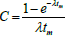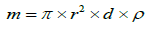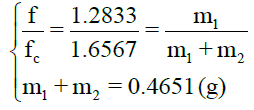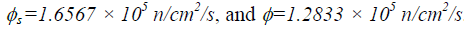All submissions of the EM system will be redirected to Online Manuscript Submission System. Authors are requested to submit articles directly to Online Manuscript Submission System of respective journal.

# Determination of Neutron Beam Diameter in 3rd Horizontal Channel of Dalat Nuclear Reactor

Nguyen AS1*, Dang L1 and Ho HT2

1Dalat University, 01 Phu Dong Thien Vuong, Dalat, Vietnam

2Nuclear Research Institute, 01 Nguyen Tu Luc, Dalat, Vietnam

*Corresponding Author:
Nguyen AS
Dalat University, 01 Phu Dong Thien Vuong
Dalat, Vietnam
Tel: (+84) 977363840
E-mail: [email protected]

Received Date: 18/04/2017; Accepted Date: 03/05/2017; Published Date: 09/05/2017

Visit for more related articles at Research & Reviews: Journal of Pure and Applied Physics

## Abstract

Neutron beam diameter (NBD) is a very important parameter in experimental nuclear in the nuclear research reactor. Accurate determination of the NBD is the best method, which makes an optimal sample size correctly, to reduce data analysis errors. To identify the NBD, we can use some methods: simulation by Monte-Carlo method, neutron imaging, and neutron activation analysis. In this report, we determined NBD of the 3rd horizontal channel of Dalat nuclear reactor that activated Gold foil. The results showed this method to be more quickly and correct results, and experimental material could reuse for another experiment.

#### Keywords

Neutron activation analysis, Neutron beam diameter, The 3rd horizontal channel of Dalat nuclear reactor, Neutron imaging.

#### Introduction

Neutrons have played an important role in the characterization, development, testing and application of materials over the past 70 years. Determination of NBD in nuclear research reactor (NRR) is necessary that build shielding system, sample sharp, sample position. The correct NBD would reduce the experimental result errors and choose better nuclear material shielding. Normally, three methods determined the NBD as the following:

- Simulation;

- Activation.

The traditional experimental method determined the NBD of the neutron channel in the nuclear reactor was the neutron radiography (NR). The first successful experiments in neutron radiography were carried out in 1935 after the discovery of the neutron, by Kallmann and Kuhn using a small neutron reactor [1,2]. Thanks to this method, the NBD could find the shape of neutron beam by a photo of the film but it was unclearly. The sharpness of the neutron beam depended on the quality of film, activated timing…. So it was the main restriction of the method.

For simulation method, in the principle, we could calculate and simulate filtered neutron, and the NBD at any neutron paths of channel of nuclear reactor [3,4]. However, comparison between the calculated and experimental results is more differential results. Therefore, the method was to be prepared before to do the real experiment, so it could not replace empirical methods. Typically, in the horizontal channel of nuclear reactor, construction of the neutron beam guide configured out, and still adjusts accordingly that must real experiment.

Recently, after setup guiding and focusing neutron beams, the experimental method often used to activate Gold foil was determined. Normally, the foil activated by two ways as the following [5,6]:

- Using activated very small gold foils, which put surrounding the neutron beam position. After gold foils were activated, measured delay gamma from small foil we could find the NBD;

- Using activated more gold strings, which arranged a grid. After finishing activation, cut the gold strings, measured delay gamma from small gold fractions we could find the NBD.

However, the limits of two ways of this method were to be measured delay gamma several times, easily mistaken position of gold foils. The error of diameter neutron depends on the size of the gold fraction strongly.

In this paper, we activated foils which are the same material (this research used gold foils, 197Au) and same activation position in the way of outcome neutron. This experiment was set up in the 3rd horizontal channel of DNR. One gold foil is smaller diameter than the NBD and another is larger diameter than the NBD. Thus, in this method, the small foil would activate whole its, and large foil would not activate whole its.

Dalat nuclear reactor (DNR) is a NRR, which based on TRIGA mark II design. It has 4th horizontal irradiation channels and thermal neutron flux (n.cm-2.s-1):

+ Tangential beam port No. 3: 1.4 × 106 (n, 2 gamma)

+ Radial beam port No. 4: 1.8 × 107 (PGNAA, NDT…)

+ Radial beam ports No. 2: 1.2 × 107 (PGNAA)

+ Radial beam ports No. 1: not used (Figure 1).

The tangential beam port No. 3 (the 3rd horizontal) of the DRN was designed to transport neutrons to the thermal neutron, where the instrument using the gamma-gamma coincidence spectrometer was installed.

The previous researches, NBD of the 3rd neutron channels of DNR determined neutron radiography. In the Figure 2, the NBD sharpness was not clearly, the border was blurred, so the value of NBD was false.

Figure 2: The sharpness of the neutron beam of 3rd channel by neutron radiography.

#### Theoretical

In the neutron activation analysis, the incidence neutron flux come to sample was determined as the following function :(1)

where Net is the net area of the photoelectric peak; tm is the counting time on second (s); A is the mass atom;is the saturation factor; tc is the irradiation time (s);is the factor decay time; td is the decay time (s);is the factor counting times; m is the weight of the activated sample (gram); I0 is gamma intensity (gamma branch ratio, %); θ is abundance isotope; εγ is efficiency of spectrometer at photoelectric gamma peak energy; σ is thermal cross section (barn); NA is Avogadro constant number,).

In the nuclear reaction, 197Au foil activated neutron, forming 198Au, then decays minus beta, and 198Hg production. 198Hg would de-excite to gamma rays emitting. In this experiment, gamma ray was selected 411.8 keV interesting (because 411.8 keV gamma ray has nearly 100% branch ratio). Figure 3 showed decay scheme of 198Au .

Because the filtered neutron was same materials whole places, and neutron flux was same value on the same horizontal cross sections, so in the horizontal distance of NBD that has same neutron flux values. Figure 4a described relative neutron flux values depended on NBD.

#### Experiment and Discussion

Experimental Description

The experiment established on the 3rd channel of DNR. Figure 4b showed the 3rd channel of DNR structure configuration.

The 3rd horizontal channel system consists of the steel hollow cylinder which has 203 mm outside diameter, and 3150 mm length. Inside the cylinder, a silicon crystal cylinder was to be filtered neutron, which was neutron thermalization; water was controlled neutron flux (open and close neutron beam); paraffin-B, lithium, cadmium was neutron collimator; concrete, lead was shielding.

The sample was activated a same place where was 4 cm distance from the end of the neutron collimator to outside and directly opposite. After the sample was activated completely and decayed, used the gamma HPGe detector spectrometer, which was 1.9 keV FWHM solution, 70% relative efficiency (1332 keV), and the count rate background was less 10 cps.

Two activated gold foils were produced by Russia, 197Au, 99.99% enrichment, 0.05 mm thickness. The small foil is 1/4 inch (0.635 cm) diameter, and 0.0078 grams, another foil are 1 inch (2.540 cm) diameter, and 0.651 grams, respectively.

Two activated gold foils were placed concentric, right angle and same position of neutron beam on the 3rd horizontal channel of DNR. Figure 5 showed the relative of NBD and foils.

Figure 5: The relative of NBD and foils description. (a) For the gold foil smaller diameter than NBD. (b) For the gold foil larger diameter than NBD.

#### Results and Discussions

Calculation the irradiation time (tc) was correctly so that it reduced neutron scattering on foil. The foil was irradiated, delayed, and measured the gamma spectrum with the appropriate time, respectively. In the gamma spectrum, as dissect above, we only interested 411.8 keV gamma ray. To minimize errors in the method, we collected the count of 411.8 keV peak more than 15,000 counts, so the statistical error of 411.8 keV peak was <1%. The neutron flux followed formula (1) for two different gold foils were shown on the Table 1.

Table 1: Some information of gold foils and some parameter results in formula (1).

Gold foil tc(s) td(s) tm(s) S D C S × D × C Cps(411.8 keV) f × 105 (n/ cm2/s)
Diameter(6.35 mm) 3565 9575 45273.54 0.0106 0.9719 0.9355 0.0096 0.3471 1.6567
Diameter(25.40 mm) 1620 57530 3838.16 0.0048 0.8426 0.9943 0.0040 6.7319 1.2833

In the results, the small foil diameter was activated for whole sample, so the value of φth calculated by formula (1) to correct, other hand, the large foil diameter was not activated, so φth calculated by formula (1) to less neutron flux correctly. The less neutron flux calculated depended to the overweight that the weight foil was not activated.

By calculating and reducing the overweight, we have correct weight of sample that was activated, and after that, we calculated accuracy the NBD.

Return on the formula (1), theoretically, if the sample diameter is not larger (smaller until equal) than NBD then the formula (1) take accurate the neutron flux, and always give the real neutron flux (the neutron flux was constant). On the otherwise, the formula (1) gave wrong neutron flux. For the same material foils, the neutron flux was calculated by formula (1) to depend on, where m is mass sample, which was a function of radius of sample as the following:(2)

Where r, d, and ρ is radius, thickness, and density of sample, respectively.

Now, interesting the large foil, imaging the large foil devised two concentric circle parts which one (inner circle) has m1 mass, and the diameter of inner circle was equal the NBD, and the other (annular) has m2 mass which was overweight mass, so the mass of large foil was m=m1 + m2. In this research, m=m1 + m2=0.4651 (g). Figure 6 showed imaging sample division.

From formula (1), we have:(3)

whereIf φc was obviously accurate neutron flux, then φc was must equalwhere m1 was above value analysis. Divided formula (3) by(4)

A system of equation for m1 and m2 as the following:(5)

The value ofwas also the neutron flux that collected by small foil,. In this experimental result, for Table 1,.

Table 1: Some information of gold foils and some parameter results in formula (1).

Gold foil tc(s) td(s) tm(s) S D C S × D × C Cps(411.8 keV) f × 105 (n/ cm2/s)
Diameter(6.35 mm) 3565 9575 45273.54 0.0106 0.9719 0.9355 0.0096 0.3471 1.6567
Diameter(25.40 mm) 1620 57530 3838.16 0.0048 0.8426 0.9943 0.0040 6.7319 1.2833

Applying for formula (5), the values of m1=m- m2=0.3603 (g). Using the formula (2), we have the final result of the inner circle diameter was d=1.968 cm, which was also accurate NBD.

The NBD error was determined by conventional errors with 3 parameter errors were: the thermal neutron cross section gold error, the efficiency detector error of 411.8 keV peak, and the statistical count error.So, when reducing the overweight of gold foil, which was not activated by this calculated method, the accurate NBD was d=1.968 ± 3.32 % (cm).

#### Conclusion

Thanks to the neutron activation analysis, NBD of the 3rd horizontal channel of DNR was identified. The method was simplified and exactly to determine the neutron beam diameter. This method was overcame limitations of other previously experimental ways which was the same activation method.

The other benefit of this method was the gold foil could use again or more than once. The reused activated gold was very simplified calculation, so the method reused experimental material.

The errors in this method were reduced until as best as possible by the counting time, the irradiation time, and the decay time adjustment.

#### Acknowledgements

The authors would like to thank the leader of Dalat Nuclear Research Institute has supported and help in this research.

#### References